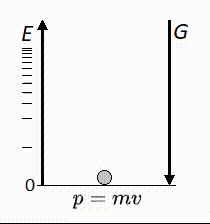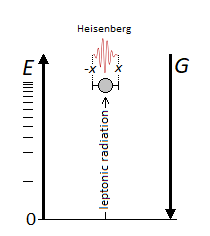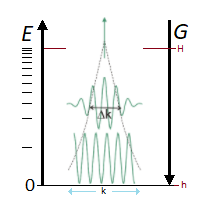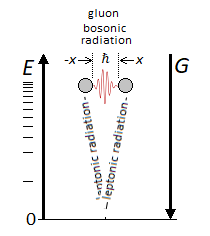# Vertical Oscillation of the Particle following the Gravitational Force Vector

## Recommended Posts

Hello.

At each departure the particle is subjected to an impulse to eject it vertically. The linear momentum decreases in relation to as it increases in height and the gravitational force that causes it to fall. How do you think we could write the forumle of momentum associated with gravitational force and on impulse? The particle is of identical mass.##### Share on other sites

Given that you have weighed in on much more complicated physics problems, one might expect you could solve a physics 101 problem

At the top of the travel the KE is zero and PE is mgH, where H is the top of the travel. The sum remains constant, so KE = mg(H-h) at all points (this assumes g is a constant)

KE = p^2/2m so the momentum will be sqrt(2m^2g(H-h))

The details of the collision aren't given; this solution doesn't apply to the impact itself and assumes a point particle (again, details are not given)

##### Share on other sites

1 hour ago, swansont said:

The details of the collision aren't given; this solution doesn't apply to the impact itself and assumes a point particle (again, details are not given)

This model does not include colision.

I now wish to associate the value of the speed into: $$\sqrt{2m^2\ g\ (H-h)}$$

Thanks.

##### Share on other sites

You will notice that the mass cancels, since the speed of a falling object is independent of mass.

##### Share on other sites

3 minutes ago, swansont said:

You will notice that the mass cancels, since the speed of a falling object is independent of mass.

Like this? $$v=\sqrt{2gh}$$

All that's missing is acceleration to finish. How is acceleration written with the same form of formula?

##### Share on other sites

34 minutes ago, Kartazion said:

acceleration to finish

What is acceleration to finish?

The acceleration is g

##### Share on other sites

Just now, swansont said:

The acceleration is g

I had forgotten that g was constant. So it doesn't work with variable acceleration.

I now wish to include Heisenberg's Uncertainty Principle in the formula. Can we write the following form? $$\Delta x\Delta p\ = \sqrt{2m^2\ g\ (H-h)} \ \ {\ge } \ {\frac{\hbar}{2}}$$

Since the projection of the particle is radiative, only one interval value of x is needed. Let x = 1 and constant. The amount of movement seems fixed. But by the principle of uncertainty, it is said that it is constraining to keep a constant value x for a macroscopic object.##### Share on other sites

34 minutes ago, Kartazion said:

I now wish to include Heisenberg's Uncertainty Principle in the formula. Can we write the following form?

ΔxΔp =2m2 g (Hh)   2

No. You need to write down the uncertainty in both the position and momentum.

You’re saying p > ℏ/2, which has the wrong units

##### Share on other sites

3 hours ago, swansont said:

You’re saying p > ℏ/2, which has the wrong units

Yes. This is because it derives from: $$\Delta x\Delta v\ \ {\ge } \ {\frac{\hbar}{2m}}$$

4 hours ago, swansont said:

What is acceleration to finish?

The acceleration is g

I meant the speed of acceleration of the particle during ejection. How to know its speed?

##### Share on other sites

5 hours ago, Kartazion said:

I meant the speed of acceleration of the particle during ejection. How to know its speed?

“Speed of acceleration”? That’s meaningless. Which one?

Speed is speed, acceleration is acceleration (which in this case is g)

##### Share on other sites

3 hours ago, swansont said:

“Speed of acceleration”? That’s meaningless. Which one?

Speed is speed, acceleration is acceleration (which in this case is g)

I am talking about the speed of the particle given by the impulse.

##### Share on other sites

3 minutes ago, Kartazion said:

I am talking about the speed of the particle given by the impulse.

Then "speed" would suffice.

p = mv, so as I said, you divide by mass and the mass term goes away. We already covered this.

##### Share on other sites

I thought I would add v to sqrt(2m^2g(H-h))

##### Share on other sites

54 minutes ago, Kartazion said:

I thought I would add v to sqrt(2m^2g(H-h))

You have mv = sqrt(2m^2g(H-h))

You need to divide both sides by m (sqrt m^2 is m)

v = sqrt(2g(H-h))

at h=H it's zero. At h = 0 it has its maximum value

##### Share on other sites

17 minutes ago, swansont said:

v = sqrt(2g(H-h))

It becomes interesting to give an interval ∆v (v2-v1), but then is it based on the interval of (H-h)?

##### Share on other sites

6 minutes ago, Kartazion said:

It becomes interesting to give an interval ∆v (v2-v1), but then is it based on the interval of (H-h)?

It would depend on h1 and h2

v2 - v1  = sqrt(2g(H-h2))- sqrt(2g(H-h1))

##### Share on other sites

3 minutes ago, swansont said:

It would depend on h1 and h2

v2 - v1  = sqrt(2g(H-h2))- sqrt(2g(H-h1))

∆v = sqrt(2g(H-h2))- sqrt(2g(H-h1))

Then everything depends on h2-h1 and time t  if x=1 for a macroscopic object with: $$\Delta x\Delta v\ \ {\ge } \ {\frac{\hbar}{2m}}$$

The time of the duration of travel of the particle gives the height traveled. The longer the time, the more uncertainty is felt.

Erratum: if x=1 then the uncertainty even for long distance is not felt.

and ∆x = (x2-x1) = 1

##### Share on other sites

14 minutes ago, Kartazion said:

∆v = sqrt(2g(H-h2))- sqrt(2g(H-h1))

Then everything depends on h2-h1 and time t  if x=1 for a macroscopic object with:

ΔxΔv   2m

What is x referring to?

If it's height, x=1 is meaningless in this context, since we haven't assigned values to anything.

∆x is an uncertainty in x, not the value of x

hbar is really, really small, so this is pretty much meaningless for such a system. If the speed and/or mass were small enough that this mattered, you would have to treat it as a wave, and then the assumption that it's a point particle isn't valid.

##### Share on other sites

Just now, swansont said:

What is x referring to?

If it's height, x=1 is meaningless in this context, since we haven't assigned values to anything.

∆x is an uncertainty in x, not the value of x

You haven't seen it because I merged it higher: ∆x = (x2-x1) = 1

2 minutes ago, swansont said:

hbar is really, really small, so this is pretty much meaningless for such a system. If the momentum of the mass were small enough that this mattered, you would have to treat it as a wave, and then the assumption that it's a point particle isn't valid.

The interest is precisely to related the particle m to the quanta.

##### Share on other sites

1 minute ago, Kartazion said:

You haven't seen it because I merged it higher: ∆x = (x2-x1) = 1

Units matter. 1 what? 1 metric tonne? 1 gram?

##### Share on other sites

7 minutes ago, swansont said:

Units matter. 1 what? 1 metric tonne? 1 gram?

Meter. x corresponds to the width of the particle or the wave packet.

If the unit is meter, then in this case it is 1 mm or 1 nm to choose from.

With the uncertainty principle, the particle oscillates along y for a width of 1 along x.

The principle of uncertainty is then real for an oscillation of magnitude, namely a greater appearance of the particle, with a decrease, or even disappearance of the visibility of the particle.

##### Share on other sites

43 minutes ago, Kartazion said:

Meter. x corresponds to the width of the particle or the wave packet.

If the unit is meter, then in this case it is 1 mm or 1 nm to choose from.

If x = 1 meter, you have a very different situation than if x is 1 nm. And it matters very much what the mass is.

43 minutes ago, Kartazion said:

With the uncertainty principle, the particle oscillates along y for a width of 1 along x.

There is only one dimension in your example. Not x and y, so that's irrelevant.

43 minutes ago, Kartazion said:

The principle of uncertainty is then real for an oscillation of magnitude, namely a greater appearance of the particle, with a decrease, or even disappearance of the visibility of the particle.

This makes no sense. Asking physics questions is fine, but if you need help solving physics 101 problems you shouldn't be making these kinds of assertions.

##### Share on other sites

7 hours ago, swansont said:

If x = 1 meter, you have a very different situation than if x is 1 nm. And it matters very much what the mass is.

I only said the unit was meter. After I specified 1 mm or 1 nm at your choices for the particle or quanta. We can also talk about 1cm. Indeed the interval Delta is x2=0,5 and x1=-0,5. But what are the different situations? You mean the principle of uncertainty which applies more or less depending on the size of the mass? This is true, because for macroscopic objects the principle of uncertainty is negligible.

7 hours ago, swansont said:

There is only one dimension in your example. Not x and y, so that's irrelevant.

You have a width of 1 for x and y which oscillates more than 1 given the height. Which makes two dimensions with a surface x*y.7 hours ago, swansont said:

This makes no sense. Asking physics questions is fine, but if you need help solving physics 101 problems you shouldn't be making these kinds of assertions.

However, it is simple if the observer is at the top at H there will be an oscillation of the particle in the form of a magnitude. As simple as that.

But then what is the unit of x here in Heisenberg's formula? $$\Delta x\Delta v\ \ {\ge } \ {\frac{\hbar}{2m}}$$

Edited by Kartazion
##### Share on other sites

It should be understood that when the particle is at h at the zero threshold and the impulse is given, then the momentum is high at the start due to its high speed up to an almost zero momentum at H, then the particle falls back. What it actually corresponds to when the impact is given, to the value of the momentum, which is equal to the k interval.We understand that the particle collapses at any time thanks to gravity, and reappears thanks to energy. If the leptonic matter is indeed radiative and vertical to gravity, then the bosons are only the difference in horizontal potential between two oscillations of the particle. It reminds me of the photon emitted by the oscillation of the electron.##### Share on other sites

Nobody?

Indeed we understand that I unite the function of gravity, to the function of the quantum particle with a demonstration 101. The universe suddenly seems less mystical.

##### Share on other sitesThis topic is now closed to further replies.
×